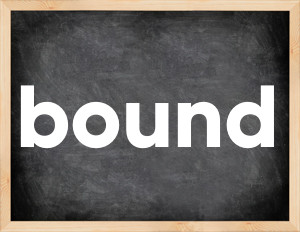# Bound past tenseThe English verb 'bound' is pronounced as [baʊnd].
Related to: regular verbs.
3 forms of verb bound: Infinitive (bound), Past Simple - (bounded), Past Participle - (bounded).

## Here are the past tense forms of the verb bound

👉 Forms of verb bound in future and past simple and past participle.
❓ What is the past tense of bound.

## Bound: Past, Present, and Participle Forms

Base Form Past Simple Past Participle
bound [baʊnd]

bounded [ˈbaʊndɪd]

bounded [ˈbaʊndɪd]

## What are the 2nd and 3rd forms of the verb bound?

🎓 What are the past simple, future simple, present perfect, past perfect, and future perfect forms of the base form (infinitive) 'bound'?

### Learn the three forms of the English verb 'bound'

• the first form (V1) is 'bound' used in present simple and future simple tenses.
• the second form (V2) is 'bounded' used in past simple tense.
• the third form (V3) is 'bounded' used in present perfect and past perfect tenses.

## What are the past tense and past participle of bound?

The past tense and past participle of bound are: bound in past simple is bounded, and past participle is bounded.

### What is the past tense of bound?

The past tense of the verb "bound" is "bounded", and the past participle is "bounded".

### Verb Tenses

Past simple — bound in past simple bounded (V2).
Future simple — bound in future simple is bound (will + V1).
Present Perfect — bound in present perfect tense is bounded (have/has + V3).
Past Perfect — bound in past perfect tense is bounded (had + V3).

### bound regular or irregular verb?

👉 Is 'bound' a regular or irregular verb? The verb 'bound' is regular verb.

## Examples of Verb bound in Sentences

•   My views were not bounded by her ideas. (Past Simple)
•   It is bounded in the south by Kenia. (Present Simple)
•   My dog came bounding to meet me. (Past Simple)
•   He bounded up when he saw me. (Past Simple)
•   Big rocks were bounding down the hill. (Past Continuous)
•   His heart bounded with joy at the sound of her voice. (Past Simple)
•   The ball struck the wall and bounded back to the boy. (Past Simple)
•   Did you know that England is bounded on the north by Scotland? (Present Simple)
•   I didn't know that the Pacific Ocean bounds California on the west. (Present Simple)
•   I am sure that you should bound your desires by reason. (Present Simple)

Along with bound, words are popular display and crack.

Verbs by letter: , , , , , , , , , , , , , , , , , , , , , , , , .